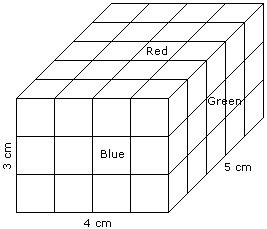# Verbal Reasoning - Cube and Cuboid - Discussion

The following questions are based on the information given below:

1. A cuboid shaped wooden block has 4 cm length, 3 cm breadth and 5 cm height.
2. Two sides measuring 5 cm x 4 cm are coloured in red.
3. Two faces measuring 4 cm x 3 cm are coloured in blue.
4. Two faces measuring 5 cm x 3 cm are coloured in green.
5. Now the block is divided into small cubes of side 1 cm each.

4.

How many small cubes will have two faces coloured with red and green colours ?

 [A]. 12 [B]. 8 [C]. 16 [D]. 20

Explanation:Required number of small cubes = 6 from the top and 6 from the bottom = 12

 Sandeep Singh said: (Mar 13, 2011) I think 12 will be answer if the question is. How many small cubes will have only two faces coloured with red and green colours ? But in above question "only" word is not used, so answer to above question should be 20. Please help me.

 Vaun Gupta said: (Jul 22, 2011) You are right Sandeep. I also think 20 is the right answer to the qs.

 Shekhar Ghate said: (Nov 6, 2011) The answer would be 20 if the question contains the phrase "at least two faces coloured with red and green". Since at least is not there hence the answer is 12.

 Anju said: (Oct 1, 2013) What is the correct answer? please explain it.

 Manish said: (Apr 1, 2015) Here nothing is written about other face colour. So it should not matter whether it is coloured or not so I think 20 is answer.

 Sikari said: (Nov 21, 2016) The answer should be 20.

 Pratham said: (Dec 2, 2016) Answer should be 20 as it is not written 'only two colours'.

 Shahwazhusain said: (Jan 29, 2017) Yeah, it should be 20.

 Srividya said: (Mar 9, 2017) Not getting it, please give the explanation clearly.

 Srividya said: (Mar 9, 2017) Please anyone give the the solution with formula.

 Annu Agrawal said: (Sep 2, 2020) According to me, the Answer is 24. 4(n1-2) + 4 (n2-2) + 4(n3-2). n = 4. 4*2 + 4*1 + 4*3 = 24.

 Bikky Kumar said: (Jan 8, 2022) According to me; 4(n1- 2) + 4(n2- 2) + 4(n3- 2) = 4(4- 2) + 4(3- 2) + 4(5- 2)=24 is the answer.